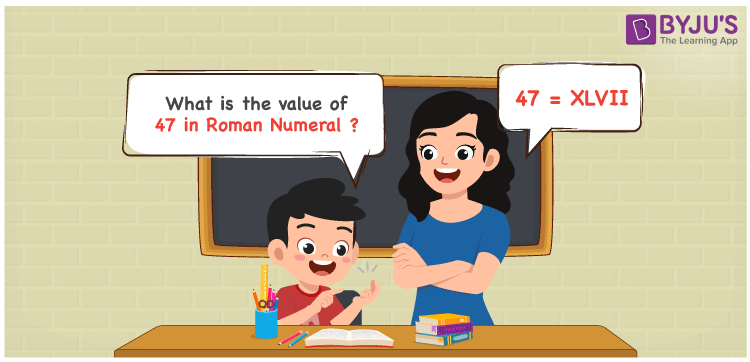# 47 in Roman Numerals

47 in Roman Numerals is XLVII. To represent any number in Roman numerals, the 7 primary symbols are used with certain rules. Refer to the rules of Roman numerals. 47 is represented as XLVII in the Roman numeral system. XLVII has both the addition and subtraction rules followed. In the representation XLVII, XL represents (the difference of 50 and 10) , V is added to the 2 times I and this result is again added to XL. Numerical representation is detailed in the next section.

 Number Roman Numeral 47 XLVII

## How to Write 47 in Roman Numerals?To convert 47 in Roman Numerals, we need to split the number according to the available symbols. i,e I, V, X, L, C, D, and M.

The simple way to split 47 will be 40 + 7. (But there are no letters for 40 and 7.)

Hence 40 can be represented as the difference between 50 and 10 and 7 as the sum of 5 and 2 ones.

So, 40 = 50 – 10 ( L – X) = XL

7 = 5 + 1 + 1

47 = (50 – 10) + 5 + 1 + 1

47 = XL + V + I + I

Therefore

47 = XLVII

## Video Lesson on Roman Numerals## Frequently Asked Questions on 47 in Roman Numerals

Q1

### How to write the number 47 in Roman Numerals?

47 in Roman Numerals is written as XLVII.
Q2

### Does VL represent the number 47?

No. VL does not represent the number 47.
Q3

### What is XLV + II in Arabic Numerals?

The Roman Numeral XLV represents 45 and II represents 2, in Arabic Numerals. So 45 + 2 = 47 or XLVII